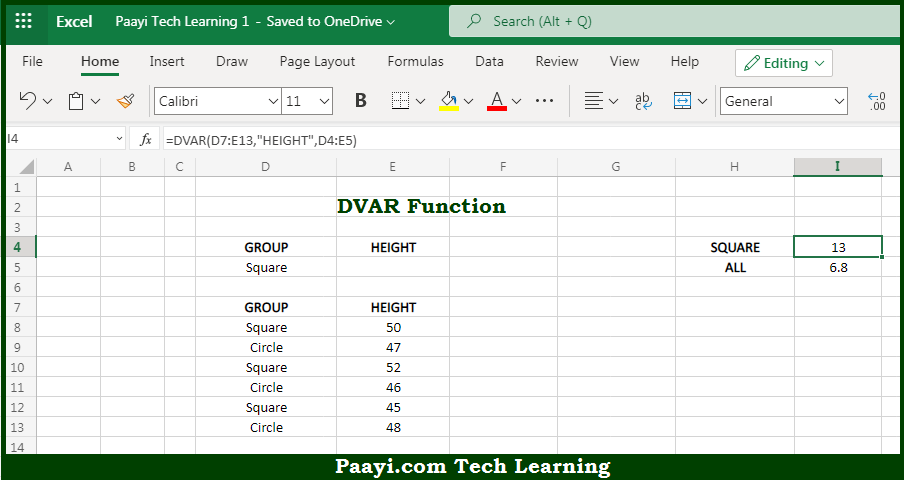# Learn How to Use Microsoft Excel DVAR Function

Written by | 0 Comments | 584 Views

In this article, you will learn how to use the Microsoft Excel DVAR function and its prime function in Microsoft Excel. You will also get to know the Microsoft Excel DVAR function return value and syntax with the help of some examples.

Microsoft Excel DVAR Function

The main purpose of the Microsoft Excel DVAR function is to get the sample variation from the matching records in the database. That implies, with the help of the DVAR function you can able to return the variance of a sample extracted from records that match the given criteria. You can use the DVARP function in case the data represents the entire population. So, with the help of the DVAR function, you can able to get the sample variation from the matching records in the database.

Return Value of DVAR Function

The return value will be the calculated variance.

Syntax of DVAR Function

=DVAR(database, field, criteria)

Where the arguments:

• database: This is the database range including headers.
• field: This is a field name or index to count optional.
• criteria: This is the criteria range including headers.

How to Use Microsoft Excel DVAR Function?So we know that Microsoft Excel DVAR function you can able to get the sample variation from the matching records in the database. That implies, with the help of the DVAR function you can able to return the variance of a sample extracted from records that match the given criteria. You can use the DVARP function in case the data represents the entire population. So, with the help of the DVAR function, you can able to get the sample variation from the matching records in the database.